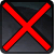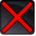# [passive_alttest]Conditions

## Related effects, buffs and debuffs

Please click on an effect below to view its details.

• [hidden] [does not expire]Effect #1

 Slot: Buff Duration: passive Tick rate: does not tick # occurrences: 0
• On Apply

Perform the following actions:

• Immobilize but allow rotation
• [hidden] [1s]Effect #2

 Slot: Buff Duration: 1s Tick rate: does not tick # occurrences: 0
• When effect expires

Perform the following actions:

• Add effect #3 to TARGET from TARGET
• Add effect #4 to TARGET from TARGET
• [hidden] [does not expire]Effect #3

 Slot: Buff Duration: passive Tick rate: 3s # occurrences: 0 Conditions: Can only by called by other effects
• When effect is applied
• When effect ticks

Only when the following conditions are met:

• If TARGET is not in combat

Perform the following actions:

• Custom
- Param 2 = (string) Pens Turret -> Combat Placeholder Agro
- Param 3 = (string)
- Param 4 = (string)
- Param 5 = (string)
- Param 6 = (string)
- Param 7 = (string)
- Param 8 = (string)
- Param 9 = (string)
- Param 1 = (int) 9499481598613081908
• Execute Hydra Hook
- Event Name = (string) Agro_Placeholder
- Object Self = (int) 1
• [hidden] [does not expire]Effect #4

 Slot: Buff Duration: passive Tick rate: 2s # occurrences: 0 Conditions: Can only by called by other effects
• On Apply

Perform the following actions:

• Unknown (183)
- Unknown (303) = (bool) true
- Unknown (306) = (bool) true
- Unknown (430) = (bool) false
- Unknown (310) = (string) aoePositionOptionType_Entity
- Unknown (311) = (string) aoeTarget_Caster
- Unknown (313) = (string) aoeScalarType_LiteralValue
- Unknown (317) = (string) aoeScalarType_LiteralValue
- Unknown (321) = (string) aoeScalarType_LiteralValue
- Unknown (325) = (string) aoeOrientationOptionType_EntityFacing
- Unknown (326) = (string) aoeTarget_Caster
- Unknown (344) = (string) aoeSphere
- Unknown (349) = (string) aoeTargetCondition_IsAlive;aoeTargetCondition_HasLOS[aoeParameter_HeightOffset:0.215]
- Unknown (353) = (string) aoeScalarType_LiteralValue
- Unknown (354) = (string) aoeScalarType_LiteralValue
- Unknown (355) = (string) aoeScalarType_LiteralValue
- Unknown (367) = (string) aoeScalarType_LiteralValue
- Unknown (384) = (string)
- Unknown (386) = (string) aoeAbilityCaster_Caster
- Unknown (387) = (string) aoeAbilityTarget_Target
- Unknown (431) = (string) aoeRotation_None
- Ability Spec = (int) 16141099150252090547
- Unknown (307) = (int) 1
- Unknown (308) = (int) 18000
- Tick Rate = (float) 2
- Unknown (304) = (float) 0
- Unknown (305) = (float) 36000
- Unknown (314) = (float) 0
- Unknown (318) = (float) 0
- Unknown (322) = (float) 0
- Unknown (362) = (float) 0
- Unknown (363) = (float) 0
- Unknown (364) = (float) 0
- Unknown (368) = (float) 50
- Unknown (390) = (float) 0
- Unknown (398) = (float) 0.1
• Perform the following actions:

• Add effect #5 to TARGET from TARGET
• Remove this effect